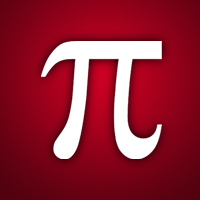Free
Overview System Requirements Related

Description

Equations is an app that helps you to solve equations, find common constants, and get common formulas. It consists of three sections: solver, constants, and formulas. SOLVER: - Equation solvers: to solve quadratic equation solvers, cubic equation solvers. - Systems Solver: to solve systems with two unknowns, three unknowns, or four unknowns. CONSTANTS: a List of most common constants. FORMULAS: more than 65 formulas, definitions and theorems in algebra, geometry, trigonometry, physics, chemistry, logarithms, and exponents.

People also like

Almohimmah

Almohimmah

12/1/2011

869 KB

For all ages

Installation

Get this app while signed in to your Microsoft account and install on up to ten Windows 10 devices.

Language supported

English (United States)